Chart Pro online
User name (email):

 Recently used

 Forex

 Indices

# Shares

Share could be defined as security that is part of the company capital, which gives the owner certain rights.

The share provides certain rights that can be classified as property rights and management rights. The rights provided by shares arising from the obligations that company has to shareholders, as defined in moment of share issuing. Liability of shareholders for the obligations of the company is limited, because shareholders take a risk for company business up to amount paid for the shares. So owners of shares cannot lose more than amount they have invested in shares.

In the case of liquidation of the company shareholder has the right to participate in the distribution of remaining assets.

Investors buy shares because they think that company will work well in the future and price of share will significantly rise. Company on the other hand issues shares to gather money for investments.

## Determining the value of shares

### Method based on the P/E ratio

The method based on the P/E ratio is one of the most common methods of determining the value of the shares. P/E ratio is calculated as the ratio of the current market value and earnings per share (EPS).

P/E ratio shows the relation between market price and its annual earnings per share. For example, for share which has a P/E = 10 market price is ten times higher then the annual earnings per share. This means that if the yield is constant, the current price represents a yield that would be achieved in next ten years. High value of P/E ratio could mean that there are great prospects for achieving high profits and that investors have a false belief that the market will stagnate or fall.

The P/E ratio should be used carefully. The high value of P/E suggests to investor that company has high development possibilities, but the high value does not automatically mean that the company has high quality. In fact, for the P/E ratio stands that if its value is small market will grow, otherwise market will fall.

### Dividend Discount Method

The method of discounting of dividends assumes that current stock price is the sum of the current value of all future dividends.

There are various methods of dividend discounting:

The general method is based on the permanence of expected dividend as the parameter for determining stock prices. If the investor buys the share with the intention to sell it after a year, the current value of share can be defined as the sum of the current value of future dividends and the market price at the time of sale:- The current value of share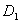- The amount of dividend that an investor will get at the end of the first year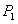- Market value of share at the end of first year- Market capitalization rate

Market capitalization rate can be calculated as the sum of dividend yield rates and rates of increase in market prices.

Dividend yield = (dividend per share) / (current market price)
The rate of increase in market prices = (increase in market price) / (current market price)

The formula for calculating the current value of over the periods (t):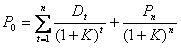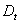- Dividend paid at the end of year t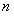- Future payments of dividends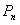- Stock price at the end of the year n

The method of zero dividend growth rate assumes that the growth rate of net gains and dividends are equal to zero. So the market value of share is determined by the formula: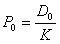- The current value of share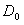- The amount of dividend that investor will receive after a year- Market capitalization rate

The method of constant dividend growth rate assumes that there is constant growth of dividends. Constant dividend growth is characteristic of companies that are in the mature stage of life cycle.

The amount of dividends that would be gained in any year (t) is calculated by the formula:- The current amount of dividend- The expected growth rate of dividend in the future

By applying this formula to the general model, equation is obtained after adjustments:Therefore, the expected rate of return is equal to the sum of dividend yield and the growth rate of dividends.

Some investments banks like Merill Linch are publishing daily calculations for many shares, and calculation is based on the dividend discount method.

### The method of free cash flow (FCF)

Free cash flow method can be applied in all those situations where the use of dividend discount method is limited: dividends are paid irregularly or not paid at all. The method is used to estimate the value of shares of companies with normal growth.

Free cash flow assumes all the company assets that remain after covering capital costs. The realized earning per share (EPS) is the maximum amount that a company could pay to shareholders in the form of dividends, so the valuation of shares by the method of free cash flow (FCF) is actually the current value of EPS.k - appropriate discount rate

If the current value of EPS is higher than share price (FCF > P), the share is oversold and we could say that this is a good moment for buying.

### Economic value added method (EVA)

Economic value added method is based on the added economic value of investment, when the price is included in the calculation of capital investment. If an investor wants to buy shares at a price P, since the profit per share belonging to each action, EVA is calculated by the formula:

EVA = EPS - KP

k - discount rate required by the owner of shares

Dividing equation with k, we get a net current value of future dividends:

The current value of future dividends = EPS/k - P

If the current value of future EVA is greater than the price of share, the share is oversold and we could say that this is a good moment for buying.